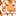# QlikView App Dev

Discussion Board for collaboration related to QlikView App Development.

Announcements
Join us at the Cloud Data and Analytics Tour! REGISTER TODAY
cancel
Showing results for
Search instead for
Did you mean:Contributor

## How to Write if condtion expression in set analysis

Hi Friends,

Can any one help me how to write if condition statement into set expression.

count(DISTINCT if((IsNull((END_DATE) and  OPEN_DATE<='2018-10-20') or  (END_DATE> '2018-10-20 'and  OPEN_DATE<='2018-10-20') ), IDs)).

in the place of 2018-10-20, I defined a variable vWeekDate.

the above expression I need to write same as by using set expression. Can any one help me on this. Thanks in advance

5 RepliesPartner
try something like below
{< ID={"=Len(END_DATE)=0"}, OPEN_DATE={"<=\$(=vWeekDate)"}> // for first and condition
+ < END_DATE={">\$(=vWeekDate)"}, OPEN_DATE={"<=\$(=vWeekDate)"}> // + for the or
}MVP

Or this

`Count(DISTINCT {<IDs = {"=(IsNull(END_DATE) and OPEN_DATE <= MakeDate(2018, 10, 20)) or (END_DATE > MakeDate(2018, 10, 20) and OPEN_DATE <= MakeDate(2018, 10, 20))"}>} IDs)`Contributor
Author

Hi Sunny,

Thank you for you reply. i have tried by using this expression like this

`Count(DISTINCT {<IDs = {"=(IsNull(END_DATE) and OPEN_DATE <= MakeDate(2018, 10, 20)) or (END_DATE > MakeDate(2018, 10, 20) and OPEN_DATE <= MakeDate(2018, 10, 20))"}>} IDs)`

i am not getting the values. but i tried belowr method like this i am getting value.

`Count(DISTINCT {<IDs = {"=(IsNull(END_DATE) and OPEN_DATE <= '2018-10-20') or (END_DATE > '2018-10-20' and OPEN_DATE <= '2018-10-20')"}>} IDs)`

I need to place a field instead of hard coded value.MVP

@shabarish0587 wrote:

i am not getting the values. but i tried belowr method like this i am getting value.

`Count(DISTINCT {<IDs = {"=(IsNull(END_DATE) and OPEN_DATE <= '2018-10-20') or (END_DATE > '2018-10-20' and OPEN_DATE <= '2018-10-20')"}>} IDs)`

Nice

@shabarish0587 wrote:

I need to place a field instead of hard coded value.

Okay, so what is the issue?Contributor
Author

Hi Sunny,

When we are using makedate function. Value is showing Zero. Same expression i am giving hard coding value . I am getting exact out put.

`Count(DISTINCT {<IDs = {"=(IsNull(END_DATE) and OPEN_DATE <= '2018-10-20') or (END_DATE > '2018-10-20' and OPEN_DATE <= '2018-10-20')"}>} IDs)`

But when we are giving makedate. it is showing Zero. So  i need to place a Date Field in the place of Hard code.